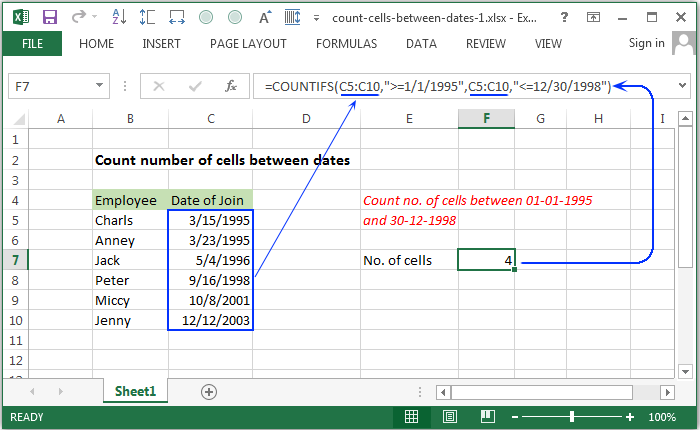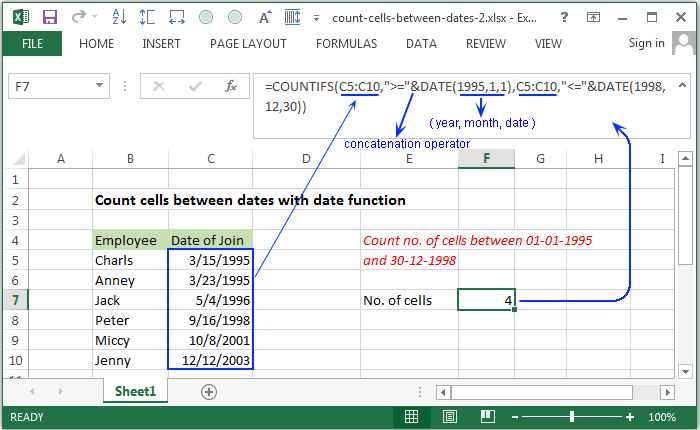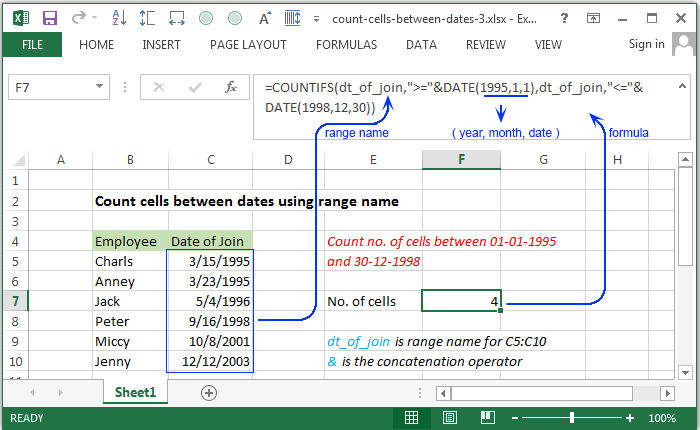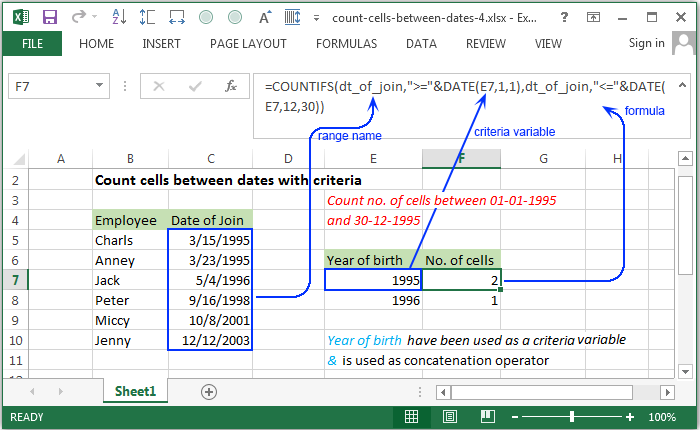# How To Count cells between two numbers

Jan 07, 2020 • edited Jan 11, 2020A quick and easy way to count between dates is by using the COUNTIFS formula. Create formula-based filters, find your sales figures, or organize your tables in no time.

Syntax of used function(s)

``````COUNTIFS(criteria_range1, criteria1, [criteria_range2, criteria2]…)
``````

Explanation

To count the number of cells that contain dates between two dates, COUNTIFS function can be used. In the following example, F7 contains this formula:

Formula

``````=COUNTIFS(C5:C10,">=1/1/1995",C5:C10,"<=12/30/1998")
``````

Copy

The above fornula contains the date of join of the employees, shown in the range C5:C10.

How this formula works

The COUNTIFS function is built to count the number of cells that matches one or more criteria. In this case, two dates have been provided. The COUNTIFS function will check whether the dates in the range C5:C10 are in the date range specified in the formula.

## Count cells between dates with date functionExplanation

To count the number of cells that contain dates between two given dates, using date function. In the following example, F7 contains this formula:

Formula

``````=COUNTIFS(C5:C10,">="&DATE(1995,1,1),C5:C10,"<="&DATE(1998,12,30))
``````

Copy

How this formula works

In this case, the date()) have been used and the year, month, and day value have been provided to form the date. The comparison operator “>=” and “<=” have been added with date function using concatinate(&) operator to make the criteria. Therefore the each date of date range C5:C11 will be searched in the newly form date.

``````DATE(1995,1,1) -> year=1995, month=1 and day=1
DATE(1998,12,30) -> year=1998, month=12 and day=30
``````

Copy

The date() makes two dates, starting and ending date of the range.

## Count cells between dates using range nameExplanation

To count the number of cells in a range name which contain dates between two given dates. In the following example, F7 contains this formula:

Formula

``````=COUNTIFS(dt_of_join,">="&DATE(1995,1,1),dt_of_join,"<="&DATE(1998,12,30))
``````

Copy

How this formula works

In this case, a range name “dt_of_join” have been introduced instead of a range of cells. The comparison operator “>=” and “<=” have been added with date function using concatination(&) operator to make the criteria. Therefore the each date from “dt_of_join” will be searched in the newly form date using date().

``````dt_of_join -> a range name for the range of cells C5:C10
DATE(1995,1,1) -> form the date 01-01-1995
DATE(1998,12,30) -> form the date 30-12-1998
``````

Copy

## Count cells between dates with criteriaExplanation

In the following example, F7 contains this formula

Formula

``````=COUNTIFS(dt_of_join,">="&DATE(E7,1,1),dt_of_join,"<="&DATE(E7,12,30))
``````

Copy

How this formula works

In this case, a range name “dt_of_join” have been used which contains a range of cells. In the date() the year part have been used as a variable. Therefore the each date form “dt_of_join” will be searched in the newly formed date range and count number of date found in the given range.

``````dt_of_join -> a range name for the range of cells C5:C10
DATE(E7,1,1) -> E7 the cell address which is used as variable for the year part of the date.
``````
#Tutorial#Count

How to Count Blank/Nonblank Cells

How To Make a Pre-Made Calendar in Excel